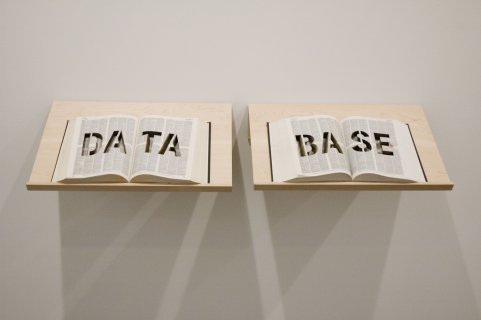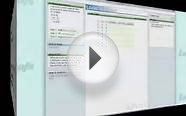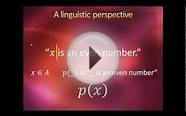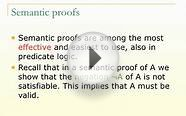# Semantics of Predicate Logic

### Definition 4 (Semantics of predicate logic - Interpretation)

An interpretation is a pair , where

• is an arbitrary nonempty set, called domain, or universe.
• is a mapping which associates to

Let be a formula and be an interpretation. We call an interpretation for , if is defined for every predicate and function symbol, and for every variable, that occurs free in .

Example: Let and assume the varieties of the symbols as written down. In the following we give two interpretations for :

For a given interpretation we write in the following instead of ; the same abbreviation will be used for the assignments for function symbols and variables.

### Definition 5 (Semantics of predicate logic - Evaluation of Formulae)

Let be a formula and an interpretation for . For terms which can be composed with symbols from the value is given by

The value of a formula is given by

The notions of satisfiable, valid, and are defined according to the propositional case (Semantic (Propositional logic)).

Note that, predicate calculus is an extension of propositional calculus: Assume only -ary predicate symbols and a formula which contains no variable, i.e. there can be no terms and no quantifier in a well-formed formula.

On the other hand, predicate calculus can be extended: If one allows for quantifications over predicate and function symbols, we arrive at a second order predicate calculus. E.g.

#### Problem 1 (Predicate)

The interpretation as follows:

Determine the value of following terms and formulae:

#### Problem 3 (Predicate)

Traffic stats
###### You might also likeLogic & Proofs: The TruthLab8.1 Predicate Logic: Symbols & TranslationLO34: Semantic Trees in Predicate Logic
Related Posts